Courses

# Short Answer Type Questions- Triangles Class 9 Notes | EduRev

## Class 9 Mathematics by Full Circle

Created by: Full Circle

## Class 9 : Short Answer Type Questions- Triangles Class 9 Notes | EduRev

The document Short Answer Type Questions- Triangles Class 9 Notes | EduRev is a part of the Class 9 Course Class 9 Mathematics by Full Circle.
All you need of Class 9 at this link: Class 9

Question 1. The angles of triangle are in the ratio 2 : 3 : 4. Find the angles.
Solution:
Let the angles be 2x, 3x and 4x.
We know that the sum of the angles is 180°.
∴ 2x + 3x + 4x = 180°
⇒ 9x = 180°
⇒ x=(1800/9)= 20°
∴ 2x = 2 x 20° = 40° 3x = 3 x 20° = 60° 4x = 4 x 20° = 80°
∴ The required angles are 40°, 60° and 80°.

Question 2. In a ΔABC, if ∠ A + ∠ B = 110° and ∠ B + ∠ C = 132°, then find ∠ A, ∠ B and ∠ C.
Solution:
We have ∠ A + ∠ B = 110°            ...(1)
∠ B + ∠ C = 132°                ...(2)
Also ∠ A + ∠ B + ∠ C = 180°             ...(3)
∴ From (1) and (3), we have 110° + ∠ C = 180°
⇒ ∠ C = 180° - 110° = 70°

Now, from (2), we have ∠ B + 70° = 132°
⇒ ∠ B = 132° - 70° = 62° From (1),
∠ A + 62° = 110°
⇒ ∠ A = 110° - 62° = 48°
Thus, the required angles are ∠ A = 48°,
∠B = 62° and ∠ C = 70°

Question 3. Prove that ΔABC is an isosceles if and only if altitude AD bisects BC.
Solution:
In ΔABC, the altitude AD bisects BC. i.e. BD = DC Now, in ΔABD and ΔACD, we have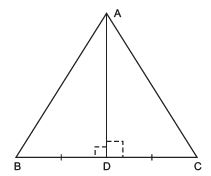BD = DC             [Given]
[each = 90°, ∵ AD is an altitude.]
∴ ΔACD ≌ ΔABD
∴ Their corresponding parts are equal.
⇒ AB = AC
⇒ ΔABC is an isosceles triangle.

Question 4. In the adjoining figure,
Find ∠ A and ∠ B.
Solution: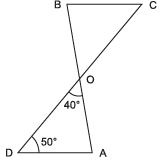∴ ∠ A= ∠ B,
Since, ∠ A + ∠ D + ∠ AOD = 180°
⇒ ∠ A + 50° + 40° = 180°
⇒ ∠ A = 180° - 50° - 40°
= 90°
∴ ∠ B= ∠ A = 90°
Thus, ∠ A = 90° and ∠ B = 90°.

Question 5. In the figure, AD ⊥ BC and AB = AC. Find ∠ B.
Solution:
∵ AD ⊥ BC and ∠ C = 55°             [Given]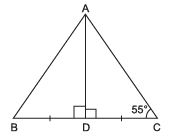Hypt. AB = Hypt. AC             [Given]
⇒ ΔABD ≌ ΔACD             [RHS criteria]
⇒ ∠ B= ∠ C
⇒ ∠ B = 55°

Question 6. In the adjoining figure, AB = BC = AC, then find the measure of ∠ A.
Solution:
∵ AB = BC = AC
∴ ΔABC is an equilateral triangle.
⇒ ∠ A= ∠ B = ∠ C
∴ ∠ A + ∠ B + ∠ C = 180°
⇒ ∠ A + ∠ A + ∠ A = 180°
⇒ 3∠ A = 180°
⇒ ∠ A =(1800/3)= 60°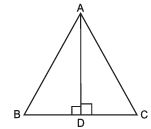Thus, the required measure of ∠A is 60°.

Question 7. In ΔABC, if ∠ A = 80°, ∠ B = 70°, then identify the longest and the shortest sides of the triangle.
Solution:
Since, ∠ A = 80° and ∠ B = 70°
∠ C = 180° - (∠ A + ∠ B)
= 180° - (80° + 70°)
= 180° - 150° = 30°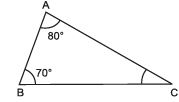⇒ Largest angle is 80°,
i.e. ∠A = 80°
∴ Side opposite of ∠A is the longest.
⇒ BC is the longest side of ΔABC
Again ∠C is the smallest angle
∴ Side opposite of ∠C is the smallest. i.e. AB is the smallest side of ΔABC.

Question 8. In the figure, ABC is a triangle in which AB = AC. The side BA is produced to P such that AB = AP. Prove that ∠ BCP = 90°.
Solution:
∵ AB = AC             [Given]
∴ The angles opposite to AB and AC are equal.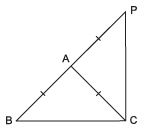⇒ ∠ ABC = ∠ ACB             ...(1)
Also, AC = AP             [Given]
⇒ ∠ APC = ∠ ACP             ...(2)
Adding (1) and (2), we get
∠ ABC + ∠ APC = ∠ ACB + ∠ ACP
⇒ ∠ ABC + ∠ APC = ∠ BCP
⇒ ∠ PBC + ∠ BPC = ∠ BCP               [∵ ∠ ABC = ∠ PBC and ∠ APC = ∠ BPC]
⇒ ∠ PBC + ∠ BPC + ∠ BCP
= ∠ BCP + ∠ BCP            [Adding ∠ BCP to both sides]
⇒ 180° = 2∠ BCP
⇒ ∠ BCP =(1800/2)= 90°
Thus, ∠ BCP = 90°.

Question 9. In the adjoining figure, O is the centre of the circle and AB is a diameter. If AC is any chord, then show that ∠ A = (1/2)∠COB.
Solution:
∵ O is the centre of the circle.
∴ OA = OC [Radii of the same circle]
Now, in ΔOAC,
∠ OAC = ∠ OCA             [Angles opposite to equal sides are equal.]
∠ OAC + ∠ OCB = ∠ COB
[∵ Exterior angle is equal to sum of interior opposite angles.]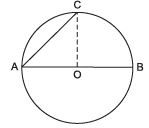⇒ ∠ OAC + ∠ OAC = ∠ COB
⇒ 2∠ OAC = ∠ COB
⇒ ∠ OAC = (1/2)∠COB
Thus, ∠ A= (1/2)∠COB

Question 10. In the figure, ABC is a triangle such that ∠ B = 40° and ∠ C = 50°. The bisector of ∠ A meets BC in X. Write AX, BX and CX in the ascending order.
Solution:
∵ ∠ B = 40° and ∠ C = 50°             [Given]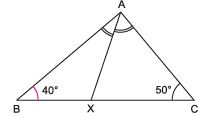∴ ∠ A = 180° - (∠ B + ∠ C)
⇒ ∠ A = 180°- (40° + 50°)
⇒ ∠ A = 180° - 90° = 90°
Since AX bisects ∠BAC.
∴ ∠ BAX = 45° = ∠ CAX
Now, ∠ BAX > ∠ ABX             [∵ 45° > 40°]
⇒ BX > AX             ...(1)
Also ∠ ACX > ∠ CAX
⇒ AX > CX             ...(2)
From (1) and (2), we have
BX > AX > CX
Thus, the required ascending order of AX, BX and CX is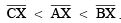Question 11. In the given figure, AD is the bisector of ∠ A of  ΔABC, where D lies on BC. Show that AB > BD and AC > CD.
Solution: In ΔABC, ∵ AD is bisector of ∠ A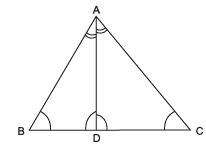In ΔABD,
⇒ AC > CD             [Side opposite to greater angle in a D is greater]
⇒ AB > BD [Side opposite to greater angle in a D is greater]

Question 12. Bisectors of the angles B and C of an isosceles triangle ABC with AB = AC intersect each other at O. Show that external angle adjacent to ∠ ABC is equal to ∠ BOC.
Solution:
In ΔABC, AB = AC
⇒ ∠B = ∠ C             [∴ Angles opposite to equal sides are equal]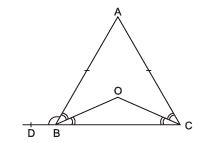⇒ (1/2)∠B = (1/2)∠C
⇒ ∠ OBC = ∠ OCB Now, in ΔBOC,
∠ BOC = 180° - [∠ OBC + ∠ OCB]
= 180° - (1/2) [∠B + ∠C]
= 180° - (1/2) [∠ B + ∠ B] [∵ ∠ B = ∠ C]
= 180° - ∠ B ... (1)
∵ ∠ ABD + ∠ B = 180°
∴ ∠ABD = 180° - ∠ B ... (2)
From (1) and (2), we have ∠ BOC = ∠ ABD
But ∠ABD is external angle adjacent to ∠ABC
Thus, [external angle adjacent to ∠ABC] = ∠BOC.

Question 13. Prove that any two sides of a D are together greater than twice the median drawn to the third side.
Solution
: In ΔABC, ∵ AD is a median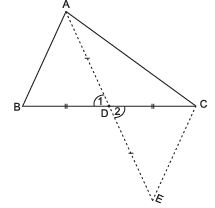∴ BD = DC
Join CE.
In ΔABD and ΔECD

BD = DC             [AD is a median]
∠ 1 = ∠ 2             [Vertically opp. angles]
⇒ ΔABD ≌ ΔECD [SAS]
∴ AB = CE             ... (1)
In ΔAEC, (AC + CE) > AE             [∵ In a D sum of any two sides is greater than the third side]
⇒ (AC + AB) > AE   [From (1)]
⇒ (AC + AB) > AD + DE
⇒ AC + AB > 2 AD
⇒ [Sum of any two sides of a D] > [Twice the median to the third side]

Question 14. In the given figure, S is any point on the side QR of ΔPQR. Prove that PQ + QR + RP > 2 PS.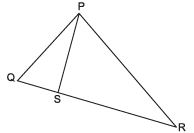Solution: ∵ In a D, the sum of any two sides is greater than the third side.
∴ In ΔPQS, (PQ + QS) > PS             ... (1)
Similarly, in ΔPRS, (PR + SR) > PS             ... (2)
[(PQ + QS) + (PR + SR)] > 2 PS
⇒ PQ + (QS + SR) + PR > 2 PS
⇒ PQ + QR + RP > 2 PS

,

,

,

,

,

,

,

,

,

,

,

,

,

,

,

,

,

,

,

,

,

;